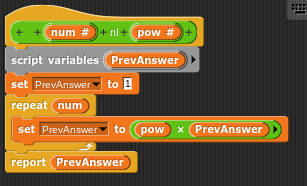# Algebraic Operators

I made a library for simple algebraic operators. I do need help with the power reporter though.

Interesting project. I wonder why all variables are named x, y, n, b, or a. That slot could just be a text input.

I was confused about the multiplication block because it looked like a function call to me. I understand that the similarity between the two glyphs x and × makes you reluctant to use the cross for multiplication, but you could use · (U+00B7), "middle dot."

Why does x have to be the denominator of the division rather than the numerator? In fact, it would be best for all the slots if the input can be a number or a variable name. You'd have to be cleverer about solving the resulting equations but it's not that hard; there are only a few cases: number number, number variable, variable number, and variable variable.

For the power block, exponentiation is repeated multiplication, not repeated addition, so that + block inside can't be right. You start with 1 and then you multiply by the first number repeating that the second number, e.g.,

set result to 1
repeat pow
set result to result x num
report result


It’s not # * x = # it’s #(x) = #
example:
2(x) = 8 (2 * 4 = 8, 2(4) = 8)
The X here is not for * (if it was I would have used the middle dot but I had no idea how to use it on non paper) the X is a variable.

It doesn’t! I just made the one way.

Also, is there a way to make a number stacked like an actual power, (6 with tiny 6 above), and also a + over - sign (+ or - used in solving for x,y,etc), and also fractions. I have done fractions (by accident) before but had no idea how?

Yes, I understand that. You want to represent a multiplication of a number and a variable. And it's true that 5(x) is a way to represent that, but it's a very unusual way. If the expression were 5(x+3) or something like that, it would look more obviously a multiplication, but nobody writes 5(x); they just write 5x. But to represent that in a block, with input slots for the pieces, I think it'd be clearer to say 5 · x. What I meant to say before is that Snap!, like Scratch, uses × to represent multiplication, but you can't do that because it'd be confused with the variable x. Using parentheses would be clearer if it were (5)(x), but 5(x) just isn't something anyone would write.

There are superscript digits in Unicode, so you can have 6⁶, but I don't see how to have an input that would use superscript digits. But you could do this:I made that block this way:so even though it looks as if base is the first input slot, really power is the first input and base the second.

It was hard for me to get Snap! to accept a space as title text. Using the regular space won't work; I gave it all the weird Unicode spaces I could find, e.g., EM SPACE (U+2003), SIX-PER-EM SPACE (U+2006), PUNCTUATION SPACE (U+2008), ZERO WIDTH SPACE (U+200B), MEDIUM MATHEMATICAL SPACE (U+205F). Most of them didn't work, but one of them, I don't know which, was actually accepted as a non-space character. And then of course title text of \$nl to force a new line before the base input.

By the way, of course the repeated multiplication algorithm works only if the power input is a positive integer. If you want a more general power function you should just use the primitive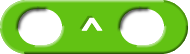block to implement it.

± is Unicode PLUS-MINUS SIGN (U+00B1).

Is this what you want for a fraction:I made the block this way:

i found a blank non-space character you could use, U+2800 BRAILLE PATTERN BLANK

I put parenthesis for people who do not know how this problem will work and don’t think 5x is something else.

Thanks you.

Thanks! (But I need the spaces:(

Edit: Did it with

(Thanks)

also you did it backwards
the script should be
[scratchblocks]
{+ + (num #) + nl + (pow #) ::operators reporter}::control hat
script variables((a)::grey)::grey
set [a v] to (pow)
repeat (num)
set [a v] to ((a) * (pow)⠀@addInput::operators)
end
report (a) :: control cap
[/scratchblocks]
edit: i did it in scratchblocks because i forgot right click > script pic existed
@earthrulerr

Thanks for the correction!

I wasn't suggesting that you use 5x; I was explaining why you never see 5(x) in the wild. My suggestion was 5 · x.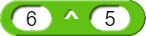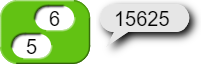It should be 7776

And a better and quicker way of calculating: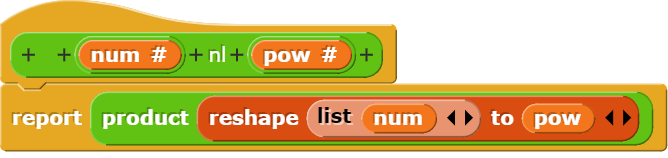In the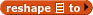block, you can ignore the list input and use a scalar value, e.g.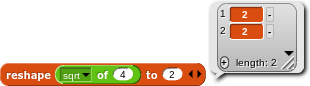okay, i didnt know that

Here's my code versus yours.

My Code: